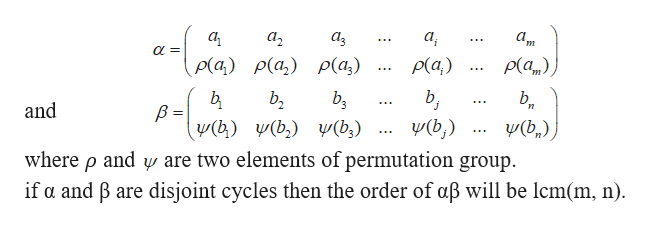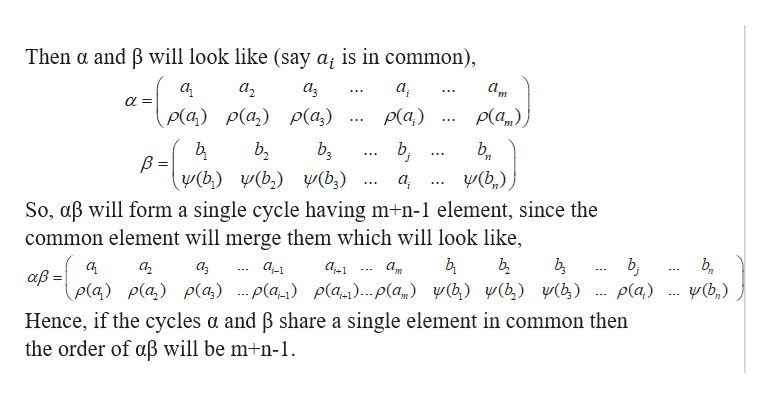# Suppose α is a single cycle of length m and β is a single cycle of length n. What can be said about the order of the element αβ if α and β are disjoint cycles? If the cycles α and β share a single element in common? If the cycles α and β share two elements in common? (There are two different answers to this last question!)

Question
240 views
1. Suppose α is a single cycle of length m and β is a single cycle of length n. What can be said about the order of the element αβ if α and β are disjoint cycles? If the cycles α and β share a single element in common? If the cycles α and β share two elements in common? (There are two different answers to this last question!)

check_circle

Step 1

The proof of third part is long. So, if you need the proof, you may re-post the particular question along with your querry.

Suppose α is a single cycle of length m and β is a single cycle of length n.

(i)   First we will observe,  that what can be said about the order of the element αβ if α and β are disjoint cycles.

Step 2

Let us denote,help_outlineImage Transcriptionclosea2 ρ(α) ρ(α,) P(a) Pla,) bg and (b)) V(b (b) (b,) (b) where p and y are two elements of permutation group. if a and B are disjoint cycles then the order of aß will be lcm(m, n) fullscreen
Step 3

(ii)   If the cycles α and β s...help_outlineImage TranscriptioncloseThen a and B will look like (say a; is in common), a a3 ρ(α) ρ(α) ρ(α) p(a,) b (b,) (b) (b)(b,) So, aB will form a single cycle having m+n-1 element, since the common element will merge them which will look like, a, a2 aß = ρ(α) ρ(α) ρ(α) ρ(α_) ρ(α). .. ρ (α,) Ψ() Ψ() Ψ(4) pla,) (b) Hence, if the cycles a and B share a single element in common then the order of aß will be m+n-1. fullscreen

### Want to see the full answer?

See Solution

#### Want to see this answer and more?

Solutions are written by subject experts who are available 24/7. Questions are typically answered within 1 hour.*

See Solution
*Response times may vary by subject and question.
Tagged in

### Math